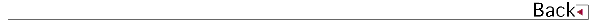\$B!!(B

# 2017\$BG/EY(B (2017.04\$B!A(B2018.03)

## \$B8&5fO@J8(B

• \$B;3K\(B \$B??G7(B, \$B@nB<(B \$B@?<#(B, \$B@>B<(B \$B9L;J(B, Facility implementation of adaptive clutter suppression to an existing wind profiler radar. First result, IEICE Communications Express

## \$B0lHL8}F,H/I=(B

• \$B@nB<(B \$B@?<#(B, \$BB@ED(B \$B905#(B, \$B2VEZ(B \$B90(B, \$B;3K\(B \$B??G7(B, \$B;V2l(B \$B?.BY(B, \$BLZ8M(B \$B9LB@(B, \$B0BED(B \$BE/(B, \$B8eF#(B \$BCi9-(B, \$B;T@n(B \$BN40l(B, \$B1+C+(B \$B=c(B, \$B:#B<(B \$BT"9/(B, \$BF#;^(B \$BH~Jf(B, \$B4d0f(B \$B9(FA(B, \$B?yC+(B \$BLPIW(B, \$B0f8}(B \$B=SIW(B,Water vapor estimation using digital terrestrial broadcasting waves, \$BF|K\CO5eOG@12J3XO"9g(B
• \$B@nB<(B \$B@?<#(B, \$BB@ED(B \$B905#(B, \$B2VEZ(B \$B90(B, \$B;3K\(B \$B??G7(B, \$B;V2l(B \$B?.BY(B, \$BLZ8M(B \$B9LB@(B, \$B0BED(B \$BE/(B, \$B8eF#(B \$BCi9-(B, \$B;T@n(B \$BN40l(B, \$B1+C+(B \$B=c(B, \$B:#B<(B \$BT"9/(B, \$BF#;^(B \$BH~Jf(B, \$B4d0f(B \$B9(FA(B, \$B?yC+(B \$BLPIW(B, \$B0f8}(B \$B=SIW(B, \$BCO%G%8J|AwGH\$NJ#?t\$NH?x5\$?dDj(B, \$B5\$>]3X2q(B2017\$BG/EY=U5(Bg2q(B
• \$B@nB<(B \$B@?<#(B, \$BB@ED(B \$B905#(B, \$B2VEZ(B \$B90(B, \$B;3K\(B \$B??G7(B, \$B;V2l(B \$B?.BY(B, \$BLZ8M(B \$B9LB@(B, \$B0BED(B \$BE/(B, \$B8eF#(B \$BCi9-(B, \$B;T@n(B \$BN40l(B, \$B1+C+(B \$B=c(B, \$B:#B<(B \$BT"9/(B, \$BF#;^(B \$BH~Jf(B, \$B4d0f(B \$B9(FA(B, \$B?yC+(B \$BLPIW(B, \$B0f8}(B \$B=SIW(B,Water vapor measurement using propagation delay of digital terrestrial broadcasting waves, 15th International Workshop on Technical and Scientific Aspects of MST Radar
• \$B?yC+(B \$BLPIW(B, \$B1+C+(B \$B=c(B, \$B%l!<%@3+H/\$H2-Fl\$N<+A3(B, \$BBh(B2\$B2s\$*\$-\$J\$o%^%j%s%5%\$%(%s%9%o!<%/%7%g%C%W(B
• \$B?yC+(B \$BLPIW(B, \$B1+C+(B \$B=c(B, \$B4d0f(B \$B9(FA(B, \$B?9K\(B \$B>\$BF|K\CO5e(B\$B!!!!!!!!!!!!(B
• \$B?9K\(B \$B>
• \$B;3K\(B \$B??G7(B, \$B@nB<(B \$B@?<#(B, \$B?yC+(B \$BLPIW(B, \$B1+C+(B \$B=c(B, \$B;38}(B \$B90@?(B, \$BCfKL(B \$B1Q0l(B, \$B%&%\$%s%I%W%m%U%!%\$%i\$K\$h\$kIwB.4QB,%G!<%?\$NIJe\$rL\;X\$7\$??.9f=hM}]3X2q2-Fl;YIt8&5fH/I=2q(B
• \$B;3K\(B \$B??G7(B, \$B@nB<(B \$B@?<#(B, \$B?yC+(B \$BLPIW(B, \$B1+C+(B \$B=c(B, \$B;38}(B \$B90@?(B, \$BCfKL(B \$B1Q0l(B, \$B%&%\$%s%I%W%m%U%!%\$%i\$K\$*\$1\$k9bJ,2rG=%G!<%?=hM}\$B!!!!!!!!!!!!!!(B
• \$B:4F#(B \$B?82p(B, \$B4d0f(B \$B9(FA(B, \$B@nB<(B \$B@?<#(B, \$B2VEZ(B \$B90(B, \$BCf@n(B \$B>!9-(B, \$B?yC+(B \$BLPIW(B, \$B1+C+(B \$B=c(B, \$B2-Fl%U%'!<%:%I%"%l%\$5\$>]%l!<%@!<\$G4QB,\$5\$l\$?@QMp1@\$N#3]3X2q2-Fl;YIt8&5f2q(B
• \$B8eF#(B \$BCi9-(B, \$B5WJ]2,(B \$B=S9((B, \$B1+C+(B \$B=c(B, \$BJ|Aw1R@1\$rCg2p\$H\$7\$?F|K\I8=`;~G[?.J}K!\$N8!F\$#3(B, \$BBh(B61\$B2s1'Ch2J3X5;=QO"9g9V1i2q(B
• \$B1+C+(B \$B=c(B, \$B?yC+(B \$BLPIW(B, \$B?9K\(B \$B> \$B!!!!!!!!!!!!!!(B
• \$B1+C+(B \$B=c(B, \$B?yC+(B \$BLPIW(B, \$B3\$LL\$K\$h\$j;6Mp\$5\$l\$?CO>e%G%8%?%k(BTV\$B?.9f\$N8!=P(B, 2018\$BG/EE;R>pJsDL?.3X2qAm9gBg2q(B
• \$BCfKL(B \$B1Q0l(B, \$B;38}(B \$B90@?(B, \$B:4F#(B \$BM*?M(B, \$B?7J](B \$BM'7<(B, \$BDZLZ(B \$BOB5W(B, \$B.@n(B \$B\$^\$j;R(B, \$B668}(B \$B9@G7(B, \$B@nB<(B \$B@?<#(B, \$BCf@n(B \$B>!9-(B, \$B4d0f(B \$B9(FA(B, \$B;3K\(B \$B??G7(B, \$BNkLZ(B \$B8-;N(B, \$BAjGO(B \$B0l5A(B, \$BNkLZ(B \$BA1=U(B, \$B5mHx(B \$BCNM:(B, \$BB-N)(B \$B%"%[%m(B, \$B]3X2q(B2017\$BG/EY=U5(Bg2q(B \$B!!!!!!!!!!!!!!(B
• \$B;3K\(B \$B??G7(B, \$B@nB<(B \$B@?<#(B, \$B@>B<(B \$B9L;J(B, \$B9bJ,2rG=(B1.3GHz\$BBS%&%#%s%I%W%m%U%!%\$%i\$N3+H/(B, \$BBh(B35\$B2s%l!<%6%;%s%7%s%0%7%s%]%8%&%`!!!!(B
• \$B;3K\(B \$B??G7(B, \$B@nB<(B \$B@?<#(B, \$B@>B<(B \$B9L;J(B, Development of a high-resolution 1.3 GHz wind profiler radar, 15th International Workshop on Technical and Scientific Aspects of MST Radar
• \$B;3K\(B \$B??G7(B, \$B@nB<(B \$B@?<#(B, \$B@>B<(B \$B9L;J(B, \$B%&%#%s%I%W%m%U%!%\$%iMQ%"%@%W%F%#%V%/%i%C%?M^05%7%9%F%`\$N3+H/(B, \$BF|K\5\$>]3X2q(B2017\$BG/EY=)5(Bg2q(B \$B!!!!!!!!!!!!!!(B
• \$B;3K\(B \$B??G7(B, \$B>pJsDL?.8&5f5!9=2-FlEE<'GH5;=Q%;%s%?!<\$K\$*\$1\$kCO5e4D6-%j%b!<%H%;%s%7%s%0\$N8&5f3+H/(B, \$BL5@~C \$B!!!!!!!!!!!!!!(B
• \$B5WJ]ED(B \$B]3X2q(B \$B=)5(Bg2q(B\$B!!!!!!!!!!!!!!(B
• \$B2H?9(B \$B=SI'(B, \$B>.EDLZ(B \$BMN;R(B, \$B?yC+(B \$BLPIW(B, \$B:4F#(B \$B9/<#(B, \$BIJ@n(B \$BM5G7(B, \$BBgLn(B \$BIR8w(B, \$BEDCf(B \$BNIOB(B, \$BG=@*(B \$B@5?N(B, \$B0f8}(B \$B@5?M(B, \$B668}(B \$B9@G7(B, \$B2#;3(B \$B2B90(B, \$B@D;3(B \$BCi;J(B, \$BCf@>(B \$BK.?N(B, Vijak Pangsapa, \$B2;GH%b!<%IBg5\$GHF0\$NCO>eHy5\$05JQF0\$K\$*\$1\$kFC@-\$HEEN%7w\$X\$N1F6A(B, \$BF|K\CO5eOG@12J3XO"9g(B
• Yusuf Jati WIJAYA, \$B5WLZ(B \$B9,<#(B, \$B?yC+(B \$BLPIW(B, Variabilities of the Sea Level Anomalies in the Upstream Areas of the Kuroshio Current, \$BF|K\5\$>]3X2q2-Fl;YIt8&5fH/I=2q(B
• \$BCf_7(B \$BM&IW(B, \$B?73@(B \$B5HLi(B, \$B@n:j(B \$BOB5A(B, \$B?{(B \$BCRLP(B, \$B@u0f(B \$BIRCK(B, \$BK-Eh(B \$B.0Q0w2q(B

## \$B9q:]2q5D4sM?J8=q(B

• \$B;3K\(B \$B??G7(B, \$B@nB<(B \$B@?<#(B, ISO/TC 146/SC 5/WG 8 \$B!I%&%#%s%I%W%m%U%!%\$%i!I(B \$B:n6H860F\$N:n@.\$KBP\$9\$k%3%a%s%H(B, ISO/TC 146/SC 5/WG 8

• \$B;3K\(B \$B??G7(B, \$B@nB<(B \$B@?<#(B, ISO/TC 146/SC 5/WG 8 \$B!I%&%#%s%I%W%m%U%!%\$%i!I(B \$B%o!<%-%s%0%0%k!<%W\$N@_N)Ds0F\$KBP\$9\$k%3%a%s%H(B, ISO/TC 146/SC 5/WG 8

## 1990\$B!A(B1991\$B!d(B1992\$B!d(B1993\$B!d(B1994\$B!d(B1995\$B!d(B1996\$B!d(B1997\$B!d(B1998\$B!d(B 1999\$B!d(B2000\$B!d(B2001\$B!d(B2002\$B!d(B2003\$B!d(B2004\$B!d(B2005\$B!d(B2006\$B!d(B2007\$B!d(B 2008\$B!d(B2009\$B!d(B2010\$B!d(B2011\$B!d(B2012\$B!d(B2013\$B!d(B2014\$B!d(B2015\$B!d(B2016 > 2017